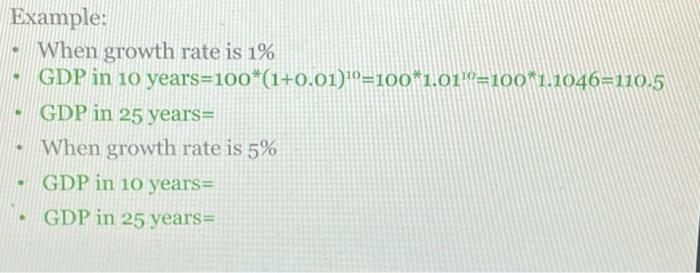### Create an Account

Home / Questions / Example: When growth rate is 1% GDP in 10 years=100*(1+0.01)10=100*1.01'0=100*1.1046=110.5...

# Example: When growth rate is 1% GDP in 10 years=100*(1+0.01)10=100*1.01'0=100*1.1046=110.5 GDP in 25 years= When growth rate is 5% GDP in 10 years GDP in 25 years

Example: When growth rate is 1% GDP in 10 years=100*(1+0.01)10=100*1.01'0=100*1.1046=110.5 GDP in 25 years= When growth rate is 5% GDP in 10 years GDP in 25 years=Jun 01 2021 View more View LessSubscribe To Get Solution